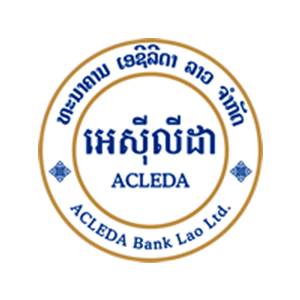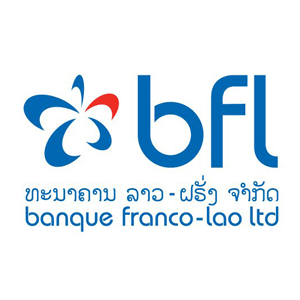Yula.la• interest 16%
• Payment period 6 years• Individual loans
• Home loans• This is testing sample
• This is testing sample
• This is testing sample
• This is testing sample
• This is testing sample
• This is testing sample• This is testing sample
• This is testing sample
• This is testing sample
• This is testing sample
• This is testing sample
• This is testing sample